# Challenge: Metronome and Cart Equations of Motion

I haven’t seen much activity on the Metronome Synchronization Challenge, so I want to provide a peak at the basis for my solution.  I took the approach of modeling a pendulum, and then linking its dynamics to the dynamics of a cart.  The challenge is choosing the right coordinate systems to assign to each of these bodies, even though they move together.

The Pendulum

If the pendulum was all by itself, we could describe its motion by modeling the angle,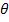, as it deflects from vertical.  The only force on the pendulum bob is gravity.  Because the pendulum is constrained to rotation about the pivot point, we only need the component of gravity in pendulum frame of reference.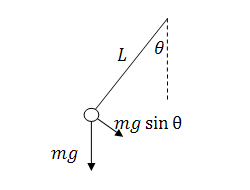If we incorporate the motion of the cart, we find the linking of the acceleration of the cart to the acceleration of the pendulum bob.  If the cart is accelerating, the reference frame of the pendulum accelerates, and this means that the pendulum will experience an opposite force/acceleration.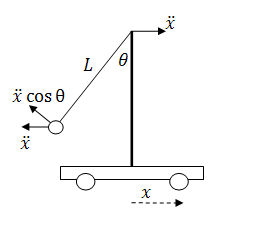Combining these two ideas in theframe centered on the pendulum pivot, we can write the pendulum equation of motion.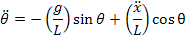Momentum in the system

To understand the acceleration of the cart, I want to look at the change in the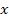component of the momentum.  Here are some definitions:

Length of the pendulum: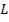Mass of the pendulum bob: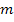Mass of the cart: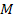The momentum of the pendulum bob in thedirection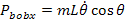The momentum of the cart is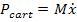The friction of the cart on the surface is the only resistance I want to model in the system, and we can describe this force as proportional to the velocity,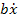. This represents of the change of momentum.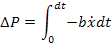Combine these terms to describe the momentum in thedirection.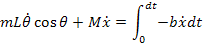Up to this point, I have looked at the problem of a single pendulum on a cart.  Adding another pendulum is just another momentum term, and to keep track of the pendulums we will introduce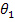and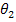.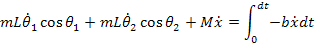Taking the derivative of this equation gives us the acceleration of the cart: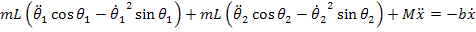System Equations

This equation for the cart, along with the dynamics of the pendulum provides the equations of motion for the system.  Rewriting these as the highest order derivatives gives us something we can create in Simulink.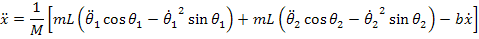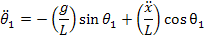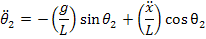Are you up to the challenge?

Can you modify the model I provided in my last post to implement these equations?  Post your solution by October 15th to the File Exchange with the keyword metronome.

|